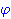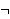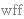New Foundations Explorer < Previous   Next > Nearby theorems Mirrors  >  Home  >  NFE Home  >  Th. List  >  wn Unicode version

Syntax Definition wn 3
 Description: Ifis a wff, so isor "not." Part of the recursive definition of a wff (well-formed formula). In classical logic (which is our logic), a wff is interpreted as either true or false. So ifis true, thenis false; ifis false, thenis true. Traditionally, Greek letters are used to represent wffs, and we follow this convention. In propositional calculus, we define only wffs built up from other wffs, i.e. there is no starting or "atomic" wff. Later, in predicate calculus, we will extend the basic wff definition by including atomic wffs (weq 1643 and wel 1711).
Hypothesis
Ref Expression
wphAssertion
Ref Expression
wnThis syntax is primitive. The first axiom using it is ax-3 7.

 Colors of variables: wff setvar class
 Copyright terms: Public domain W3C validator